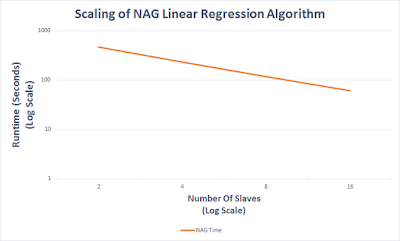This is a brief summary of a talk I gave recently at the Chicago Apache Spark Users Group Meetup. During the talk, I present many of the problems and successes when using the NAG Library distributed on Apache Spark worker nodes. You can find the slides available here.

### The Linear Regression Problem

In this post we test the scalability and performance of using NAG Library for Java to solve a large-scale multi-linear regression problem on Spark. The example data ranges from 2 gigabytes up to 64 gigabytes in the form of

 label x1 x2 x3 x4 68050.9 42.3 12.1 2.32 1 87565 47.3 19.5 7.19 2 65151.5 47.3 7.4 1.68 0 78564.1 53.2 11.4 1.74 1 56556.7 34.9 10.7 6.84 0

We solve this problem using the normal equations. This method allows us to map the sum-of-squares matrix computation across worker nodes. The reduce phase of Spark aggregates two of these matrices together. In the final step, a NAG linear regression routine is called on the master node to calculate the regression coefficients. All of this happens in one pass over the data - no iterative methods needed!

Example output from the linear regression is the following:

Time taken for NAG analysis: 236.964 seconds

Number of Independent Variables: 5

Total Number of Points: 334800000

R-Squared = 0.699

 Var Coef SE t-value Intcp 12723.3 2.2 5783.3 0 989.7 0.02 47392.5 1 503.4 0.04 11866.5 2 491.1 0.1 4911.9 3 7859.1 0.9 8732.3

*************************************************

Predicting 4 points

Prediction: 57634.9 Actual: 60293.6

Prediction: 32746.6 Actual: 35155.5

Prediction: 49917.5 Actual: 52085.3

Prediction: 82413.2 Actual: 82900.3

Timings

Using the above method, the NAG linear regression algorithm is able to compute the exact values for the regression coefficients, t-values, and errors. Below is a log-log plot of the Runtimes vs. Size of Input data for the NAG routines on an 8 slave Amazon EC2 cluster.Not only is it important the NAG routines run fast, but that they scale efficiently as you increase the number of worker nodes. Let's look at how the 16 GB data set scales as we vary the number of slaves.Comparison To MLlib

We tried running the MLlib Linear Regression algorithm on the same data, but were unable to get meaningful results. The MLlib algorithm using the stochastic gradient descent to find the optimal coefficients, but the last stochastic steps always seemed to return NaNs (we would be happy to share sample data ...let us know if you can solve the problem!).